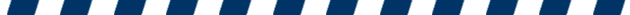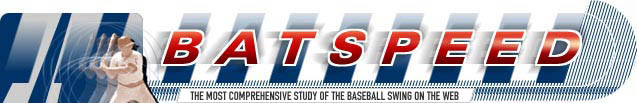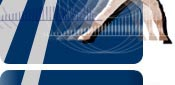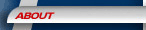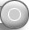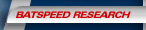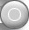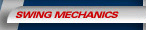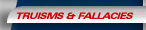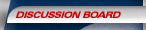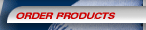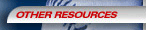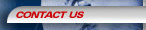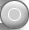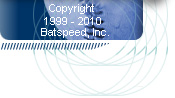Epitrochoid Hypothesis

Posted by: Al_Oha (p3d0n6@hawaii.rr.com) on Sat Jan 10 18:01:21 2009

Consider this: Bat motion can be described in the path of an epitrochoid. It is a curve in a family of many that can be created by a child's toy called a spirograph (1). It has practical application in workings of the stator and rotor of a Wankel engine (2). In fact, the "double pendulum" Mankin often refers to, is motion through an epitrochoid (3). If true, I submit that it yields a key to rotational hitting. Hand torque and circular hand path
can be rigorously analyzed and interpreted.

The epitrochoid is mathematically defined in a trigonometric function (4): The parallels to Mankin's concepts are rather compelling.

http://en.wikipedia.org/wiki/Epitrochoid

1. Hand torque is represented by motion in a circle with radius "r". In the same way, the wrists and hands go around the axis of the body in CHP (circular hand path), with the larger radius "R". The parameter "d" represents bat length.

2. A graphic can be generated as a function of time, in the full rotation in a cycle of "R". In this way, a rosette is described. Any number of "petals" or "cusps" emerge depending on the radius "r", or on the value of "d". In baseball, bat length "d", equals if not exceeds the radius of "r" and "R". The resulting curve describes one "cusp" and perhaps the makings of another. I propose that the principal "cusp" depicts what Mankin ascribes to as the "protractor"-like circle in the batpath of a swing in his DVD (5).

3. A "cusp" can be subject to geometric analysis. The derivative of any point along the curve produces the "slope" of the curve at that point. This gives the velocity if distance along the curve is measured as a function of time. The second derivative at this same
point calculates a change in velocity. In kinetic terms, this is defined as acceleration.

4. From this, there is a certain range in the value of the angle "theta" in which velocity rapidly increases, and where acceleration peaks along the upslope in the path of that
"cusp". What is evident here, is that there is no change in neither velocity in the individual rotation of CHP or torque. Nor does the slope at any point represented by either circle approach the magnitude of the "cusp's" true "synergy" is created when a value
exceeds what any comparable value of its respective components can do alone . In the Mankin framework, this fits with what has been said all along, that hand torque augments the rotation of the bat about the body's axis with a "whip" or acceleration that CHP nor
hand torque can achieve by themselves.

5. There are concepts that can be mapped onto this formulation. For example, the range in the angle "theta"through which kinetic parameters reach a crescendo, correlates to Mankin's concept of "linkage" I will refer to this as the "prime angle of theta" or theta prime." During this period, high level hitters achieve mechanics that Mankin prescribes as the "absolutes." This is the phase when the rear elbow locks "into the slot" in the power "L" position. This is the phase in which the leading shoulder begins to exerts its influence on the "L" of the leading elbow transfixed in the radius of CHP. In this phase, the interplay between top hand/ bottom (THT/BHT) hand is sustained from its inception in
the swing.

6. Paul Nyman's simulation offers some value in batswing physics (6). However, I believe his physical and kinetic modeling is "hamstrung" by its premises. What if we "think out of the box" for the moment. Consider CHP and hand torque as independent
processes, or as two separate "generators" of force. Add to this the consideration that each might occur in separate spatial domains, and not necessarily limited to the X-Y coordinates of the Cartesian plane.

7. I propose that hand torque undergoes "precession." (7). This is a change in the direction of the axis of a rotating object: If the two hands on the bat represent opposite points on a diameter of a turning wagon wheel, the points will transition through space .
These become corresponding points in the spinning diameter of a roullette table by the time the hands makes contact with the ball. That is, the axle of that wheel situated parallel to Earth, will tip skyward. As the two hands continue their motion, the axis will
deflect in the direction of the hub of the roullete table that is now perpendicular to the same surface. (The axis of a spinning top will "wobble" as it loses momentum in its fall, is another example of precession, except that the "tipping "would be in the reverse from
roullette hub to wheel axle).

The wrists/hands indeed undergo a gyration in space (8). Each starts one stacked onto top of the other at the initiation of the swing. This culminates in a "palm-up, palm-
down" relationship just before ball contact. What is evident here, is that motion at the tip of the bat follows a path in the three dimensions of space. The trajectory is not an epitrochoid, but follows a helix, or a curve in three dimensions. Suffice it to say, that this too, can be mathematically derived and kinetically analyzed. I will venture to also predict, that in precession, gravity may impart an effect however small or significant to
supplant the work accomplished by hand torque.

I contend that Nyman simulations do not adequately model hand torque as it occurs in space, to account for such elements as precession. In his models, he treats the "second pendulum" as a passive appendage when in fact it is a active system onto itself.

8. The crux of this discussion follows: In the "prime angle of theta" with respect to "R", an important fusion occurs. Hand torque at swing' s inceptions "slingshots" if you will, the bat tip with a certain velocity in the domain of space. It gets elaborated on by effects of gravity and precession. Hand torque becomes "one" with circular hand path when its plane "couples" with the plane in CHP. At "theta prime", we now have "linkage" in Mankin's terminology. "Precession" ceases, but THT/BHT continues. The trajectory of hand torque now assumes the radius of "R." A synergy is created so that in the unified system, the product manifests in bat tip kinetics powered by a circuit in the epitrochoid.

I am not a physicist or engineer. But I believe I understand enough conceptually to consider the shortcomings in what has been presented in the past in unsatisfactorily discounting or supporting Mankin's concepts. I hope this hypothesis provides some foundation for further testing and review. I will also leave how all of this gets translated into better mechanics to your experts.

1. Spirograph: http://en.wikipedia.org/wiki/Spirograph
2. Wankel Engine: http://en.wikipedia.org/wiki/Wankel_engine
3. Double Pendulum:
http://www.maths.tcd.ie/~plynch/SwingingSpring/doublependulum.html
4: Epitrochoid: http://en.wikipedia.org/wiki/Epitrochoid
5. Mankin CHP: http://www.125west.com/Batspeed_Final_Arc_II.html
6. Paul Nyman Simulation: See Setpro.com series:
http://www.batspeed.com/messageboard/292433.html

http://www.batspeed.com/messageboard/192040.html

7. Precession: http://en.wikipedia.org/wiki/Precession
8. Teaching THT/BHT: http://www.batspeed.com/messageboard/222657.html

Followups:

Post a followup:
Name:
E-mail:
Subject:
Text:

Anti-Spambot Question:This famous game is played during the middle of the MLB season?    Super Bowl    World Series    All Star Game    Championship

[   SiteMap   ]